Learn all Concepts of Linear Equations Class 9 (with VIDEOS). Check - Linear Equations in 2 Variables Class 9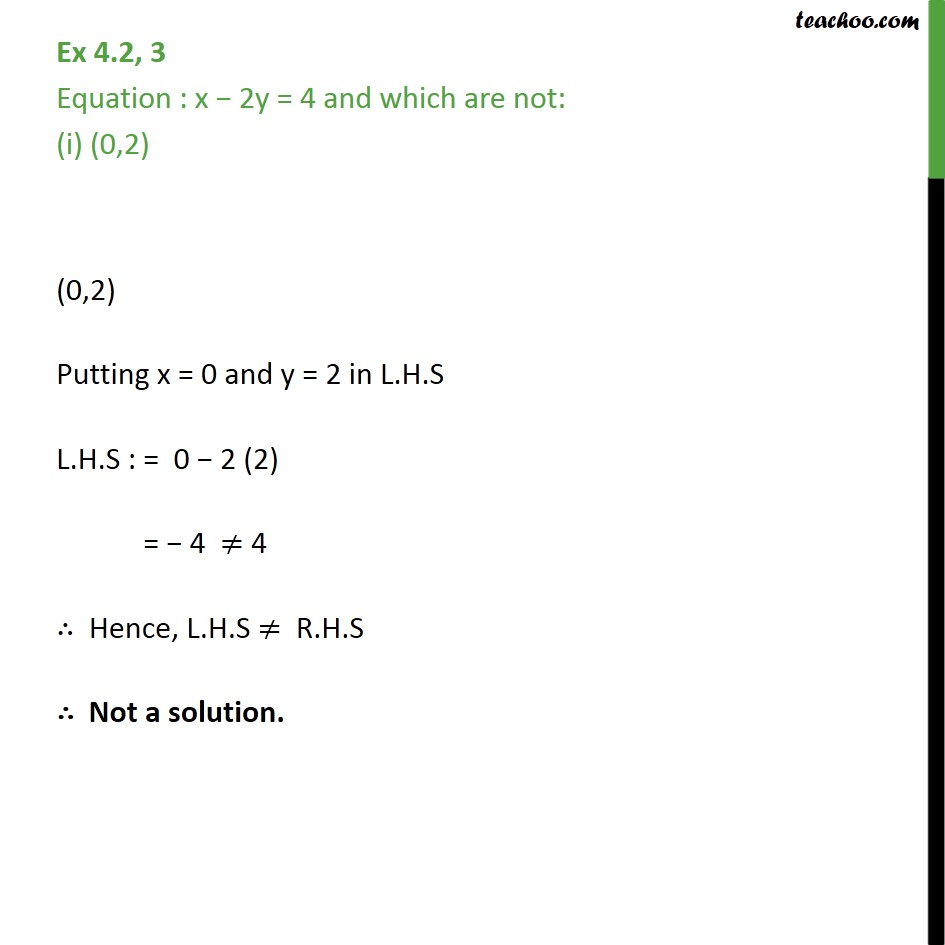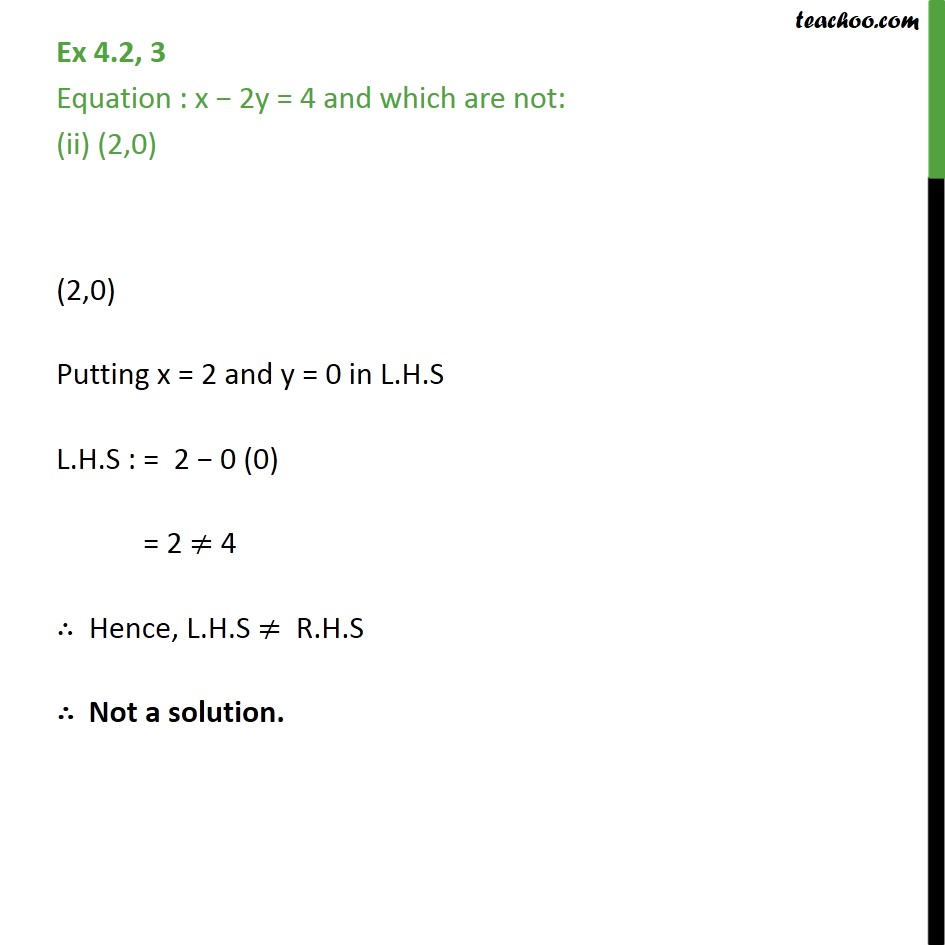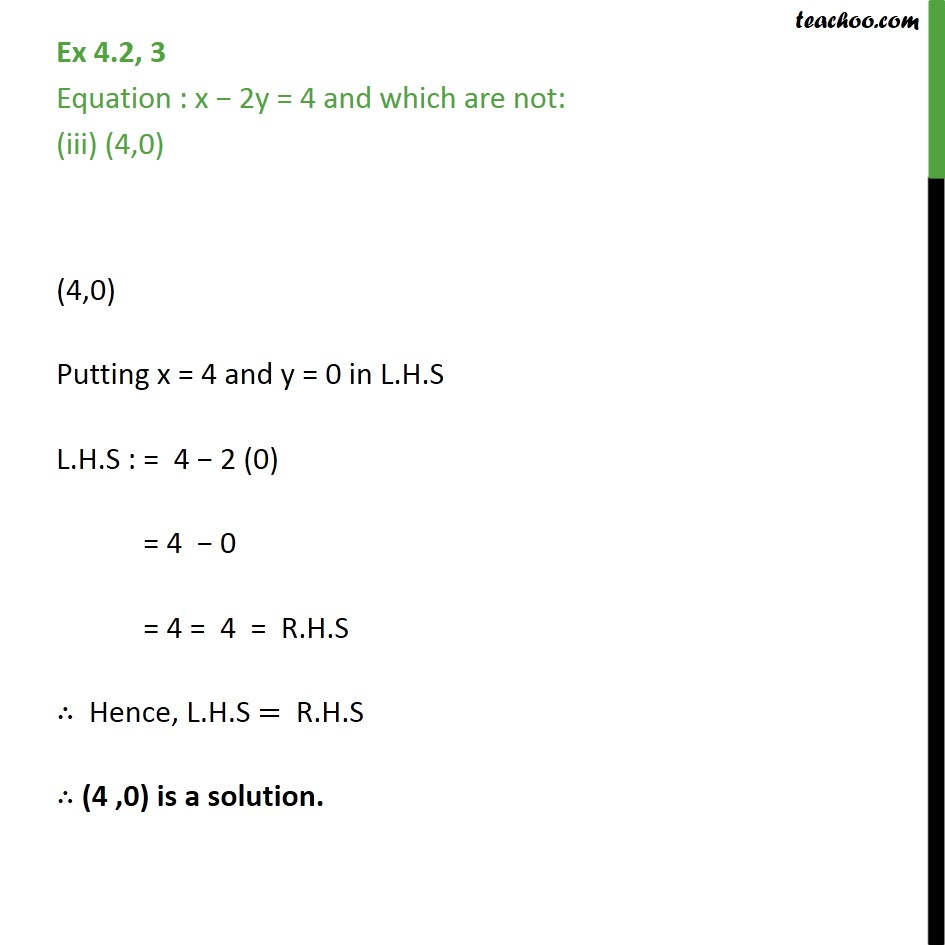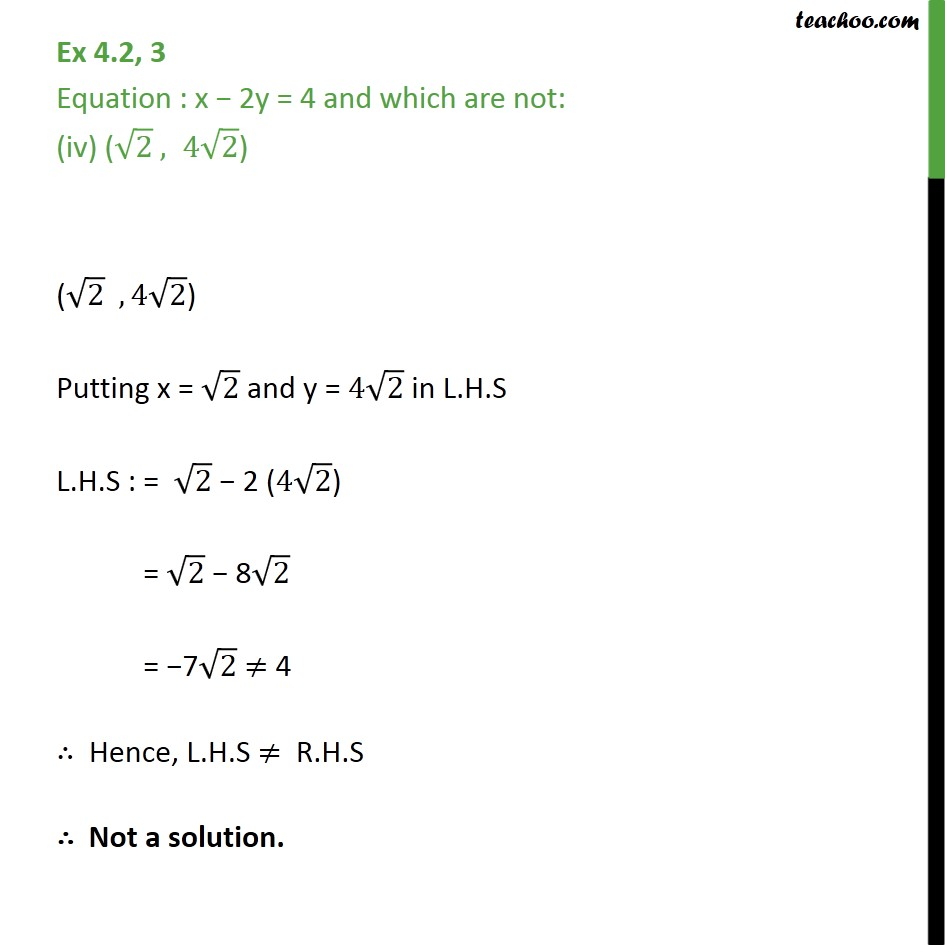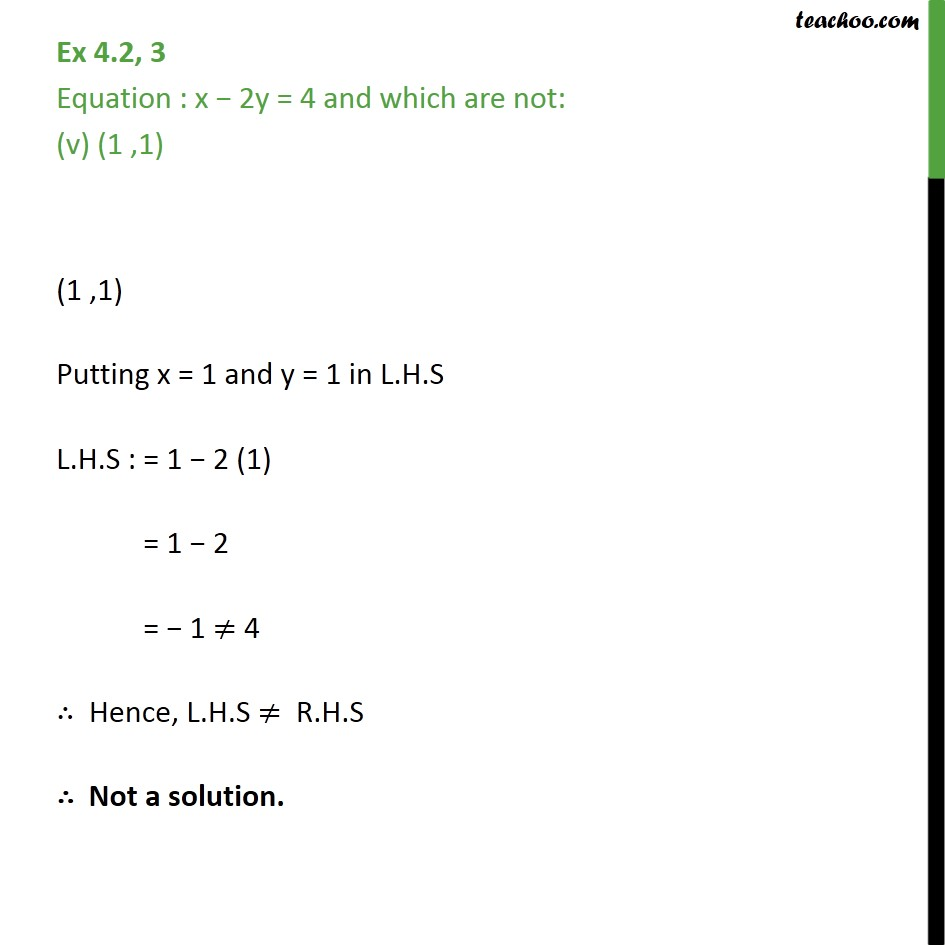1. Chapter 4 Class 9 Linear Equations in Two Variables
2. Serial order wise
3. Ex 4.2

Transcript

Ex 4.2, 3 Equation : x − 2y = 4 and which are not: (i) (0,2) (0,2) Putting x = 0 and y = 2 in L.H.S L.H.S : = 0 − 2 (2) = − 4 ≠ 4 ∴ Hence, L.H.S ≠ R.H.S ∴ Not a solution. Ex 4.2, 3 Equation : x − 2y = 4 and which are not: (ii) (2,0) (2,0) Putting x = 2 and y = 0 in L.H.S L.H.S : = 2 − 0 (0) = 2 ≠ 4 ∴ Hence, L.H.S ≠ R.H.S ∴ Not a solution. Ex 4.2, 3 Equation : x − 2y = 4 and which are not: (iii) (4,0) (4,0) Putting x = 4 and y = 0 in L.H.S L.H.S : = 4 − 2 (0) = 4 − 0 = 4 = 4 = R.H.S ∴ Hence, L.H.S = R.H.S ∴ (4 ,0) is a solution. Ex 4.2, 3 Equation : x − 2y = 4 and which are not: (iv) ( ﷮2﷯ , 4 ﷮2﷯) ( ﷮2﷯ , 4 ﷮2﷯) Putting x = ﷮2﷯ and y = 4 ﷮2﷯ in L.H.S L.H.S : = ﷮2﷯ − 2 (4 ﷮2﷯) = ﷮2﷯ − 8 ﷮2﷯ = −7 ﷮2﷯ ≠ 4 ∴ Hence, L.H.S ≠ R.H.S ∴ Not a solution. Ex 4.2, 3 Equation : x − 2y = 4 and which are not: (v) (1 ,1) (1 ,1) Putting x = 1 and y = 1 in L.H.S L.H.S : = 1 − 2 (1) = 1 − 2 = − 1 ≠ 4 ∴ Hence, L.H.S ≠ R.H.S ∴ Not a solution.

Ex 4.2